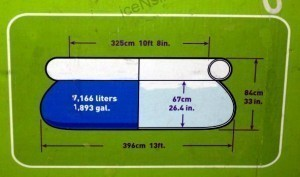•• # Liters To Gallons

One US gallon is equal to 3.78541178 liters. 1 liter is equivalent to 0.264172052 US gallons.To convert liters to gallons, multiply the figure by 0.2642. To change gallons to liters, multiply it by 3.7853.

### US Gallons and Liter Equivalents

1 US gallon is equal to 3.785 liters. 2 US gallons are equal to 7.571 and 3 gallons is the same as 11.356 liters. 4 gallons is equal to 15.141 liters and 5 gallons to 18.927 liters.

6 US gallons is the same as 22.712 liters, 7 gallons is equal to 26.497 liters and 8 gallons 30.282 liters. 9 US gallons are equal to 34.068 liters and 10 gallons is the same as 37.853 liters.

### The US Gallon

The figures given for converting liters to gallons used the US liquid gallon. However there are other gallons in use today. The abovementioned US gallon (usually rounded off to 3.8 liters) is the most commonly used.

But there is also the dry US gallon (equal to 4.4 liters) and the Imperial gallon (UK) (4.5 liters). The Imperial gallon is unofficially employed in the UK, Ireland and Canada. The gallon is also used in other (mostly English speaking) nations.

### Facts about the US Gallon

The US gallon is equal to 231 inches and 3.785411784 liters. This is also equivalent to 0.133680555 cubic feet. A fluid ounce is equal to 1/128th of the US gallon.

The US dry gallon on the other hand, is 1/8 of the US Winchester bushel (2150.42 cubic inches). When converting liters to gallons, remember that the US dry gallon is equal to 268.8025 cubic inches or 4.40488377086 liters.

### The Imperial Gallon

This gallon is equal to 4.54609l liters. Besides the countries mentioned earlier, it is still used in some Commonwealth nations. The Imperial gallon is calculated based on a volume of 10 lbs and stored at 62 F.

The US liquid gallon on the other hand, comes in at 8.3 lbs at the same temp. An imperial fluid ounce is equal to 1/160th of an imperial gallon.

### Usage

The US gallon is still utilized to measure fuel in the US and the following countries: Dominican Republic, Colombia, Haiti, Ecuador, Peru, Panama and others. The UK gallon is used for measuring oil usage (liters for every 100 km). This is why knowing how to convert liters to gallons is crucial; countries use different measurements.

### The Liter

The liter or litre is utilized in the SI measurements (although not officially part of it). It measures 0.001 cubic meters. A liter is roughly equal to 1 US quart. One US gallon is equal to about four quarts or eight pints.

A measured cup is equal to about 250 ml while a teaspoon is equivalent to 5 ml. 1 tablespoon is equal to 15 ml and the liter is equal to a cube with sides of 10 cm. This is also equal to a cube with 4 inch sides.

The need to convert liters to gallons is essential. But as you can see, the conversion process isn’t that hard.